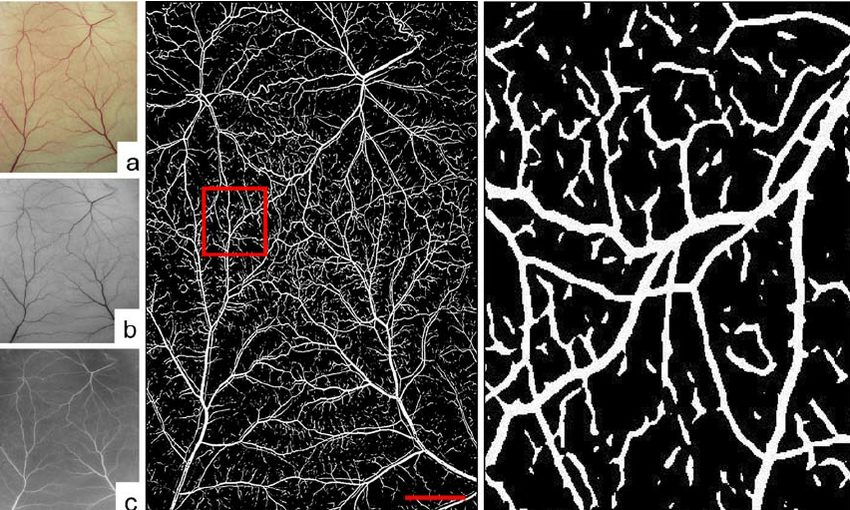## Image Processing

Image analysis is performed using a variety of algorithms and methods. Some techniques are more effective than others and usually depend on the particular application. These algorithms can be classified many ways into various logical groups which can then be combined and grouped further into application sub groups. The two major logical groups being employed in this work are segmentation and edge detection which combine to create various methods for object tracking.

## Segmentation

Segmentation is the process of dividing an image based on shapes and pixel intensity values. If the algorithm uses both shape and intensity values to classify and segment, then it is known as Contextual Segmentation whereas if only intensity values are used, it is known as Non-Contextual Segmentation.
A frequently used example of contextual segmentation is Delaunay Triangulation. This algorithm is better recognized in the field of finite elements, but has related uses in the field of image classification as well. It is a triangulation of a set of points such that no point inside the circumcircle of any triangle lies within the set of points. It attempts to maximize the interior angles of the triangles i.e. it is the nerve of the cells in a Voronoi diagram. Remi and Bernard discuss in their paper a generic tracking algorithm based on Delaunay Triangulation that effectively tracks objects without needing a specific model to be initially defined, making it adaptable to work with other applications.
Used extensively throughout this research, thresholding is a prime example of noncontextual segmentation and is the basis for background removal. Accurate and efficient extracting of moving objects for detection and tracking relies on efficient background elimination and noise removal. Segregating the background from the foreground is accomplished by setting a threshold for the differences between two frames, typically of the same setting and at different times. By overlaying the frames and performing a pixel by pixel subtraction, pixels of the target frame whose difference in intensities from the reference frame fall below a certain difference threshold are considered to be the same in both frames and therefore to be part of the background while the target frame pixel is set to zero. Any set of pixels whose difference is above the threshold is considered to be part of the moving foreground and the corresponding pixels in the target frame are either unchanged or set to one, depending on the desired application. This subtraction and comparing of pixels is known as frame differencing, and while there are various algorithms for background removal, they all use this as a basis.
In the vast majority of cases, the background will not remain entirely unchanged. For instance, furniture gets moved, items initially not in the frame come in and are left, etc. To account for these changes, the reference frame should continuously be updated after a given amount of time so that excess noise can be minimized. This updating of the background frame is known as adaptive background subtraction. This method has been shown to be more effective and efficient at locating the foreground and minimizing background noise. Even using modified differencing methods like adaptive background subtraction, some noise usually still exists and therefore a filter needs to be applied to obtain the desired isolated image. Three commonly used filters include Gaussian, mean, and the one used in this research, the Wiener filter.
The Gaussian filter is a low pass filter that modifies the input signal by convoluting it using the Gaussian function. This method is also known as the Weierstrass Transform after Karl Weierstrass. The Gaussian distribution is a standard bell curve which plays an important role in its application to filtering as it defines a probability distribution for noise. When applying the function to images, instead of being only one dimensional, it needs to be two dimensional; this is just the product of two one dimensional Gaussian functions, one in each direction. Drawbacks of using the Gaussian filter are that because it works by smoothing an image, it is not effective at removing what is known as salt and pepper noise, the random black and white specs that appear can appear in images, nor does it typically maintain the brightness of the original image.
The mean filter is much more effective at reducing this salt and pepper noise as it slides over each pixel and replaces its value with the mean of the surrounding pixels. This surrounding area is determined from an initial value preset by the user. Depending on the application, the typical noise amount present, and the desired effectiveness, different size windows are chosen. Similar to the mean filter is the median filter which replaces each pixel value with the surrounding area’s median value rather than its mean. An attractive advantage of using a median filter, rather than a mean filter, is that it is better at preserving the details of the original image Chosen for this research, the Wiener filter, named for Norbert Wiener, is a linear filter that minimizes the mean square error of the desired response and the actual output of the filter. The minimum of the mean square error occurs when the correlation between the signal and the error is zero. Mathematically, this is when the two signals are said to be orthogonal, meaning the dot product of the two is equal to zero. The Wiener filter is frequently used in deconvolution which leads to many applications in image processing, especially for adaptive background suppression.segmentation in image processing